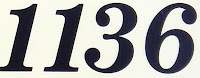## Monday, October 17, 2011

### 1136

1136 = 24 x 71.

1136 is a divisor of 1710 - 1.

1136 is a member of the toothpick sequence starting from a cross formed from four toothpicks.

1136 is the number of ways of writing 4 as a sum of eight squares.

1136 is the smallest number whose sum of squares of digits is 47: 12 + 12 + 32 + 62 = 47.Spanish mathematician and astronomer Abraham bar Hiyya Ha-Nasi (Savasorda) died in the year 1136.